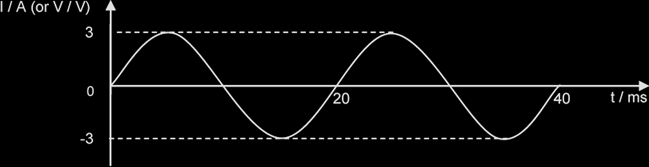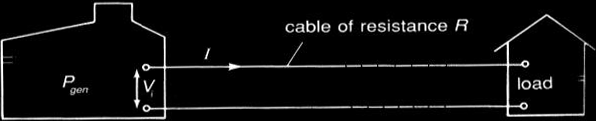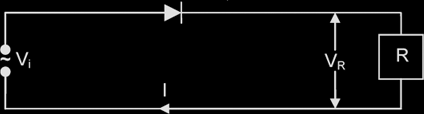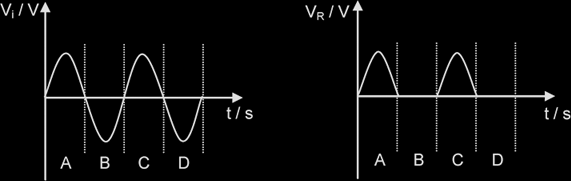# Alternating Currents

#### Characteristics of alternating currentsPeak current, I0 = 3 A

Peak-to-peak current, Ip-p = 6 A

Period, T = 20 ms

Frequency, f = 1 / T = 50 Hz

Angular Frequency, ω = 2 π f = 314 rad s-1

Instantaneous current: the current at a particular instant.

Since this A.C. signal can be described by the equation:
I = I0 sin (ω t)
or V = V0 sin (ω t)
the instantaneous current I or voltage V at time t is given by I0 sin (ωt) or V0 sin (ωt).

Note: Both the period and amplitude of a sinusoidal A.C should be constant.

Root-mean-square current of an alternating current is defined as that steady {NOT direct} current that produces the same heating effect {ie I2 R} as the alternating current in a given resistor.

(Instantaneous) sinusoidal current: I = I0sinωt , { Similarly, V = V0 sinωt }

Irms = Io / √2, Vrms = vo / √2, {for sinusoidal ac only}

Relationship between Peak, & RMS values of PD & Current: V0 = I0R , Vrms = IrmsR

Mean/Ave Power, Pave = Irms2 R = Vrms2 / R = Irms / Vrms = ½ x Maximum Instantaneous Power = ½ I0V0 {for sinusoidal AC}

Max (Instantaneous) Power, Pmax = I0V0 = I02 R

The root-mean-square (R.M.S.) value, Irms, of an A.C. is the magnitude of the direct current that produces the same average heating effect as the alternating current in a given resistance whereas peak value is the maximum current of an AC.

Ideal transformer: Vp Ip = Vs Is → NS / NP = VS / VP = IP / IS

{Mean power in the primary coil = Mean power in the secondary coil )

{Values of I & V may be either R.M.S. or peak but not instantaneous values; NS / NP: turns ratio}

#### Power Loss during Transmission of Electrical PowerPower Generated at power station Pgen = Vi I,
where I: current in the transmission, Vi: Voltage at which power is transmitted

I = Pgen / Vi

Power Loss in Transmission Cables, PL = I2 RC = (Pgen / Vi)2 RC ;RC = cable resistance

Thus to reduce power loss, for a given amt of power generated, electricity is transmitted at high voltage Vi {ie low current}. {Vi is NOT the pd across the cables}

#### Rectification with a diode

If a single diode is connected to an A.C. circuit as shown, a half-wave rectification occurs.The graphs for the input and output voltages, and the output current, are shown below.In the regions A and C, the diode is forward biased, allowing current to flow. When the input voltage becomes negative, the diode prevents the current flow, because it is reverse biased.